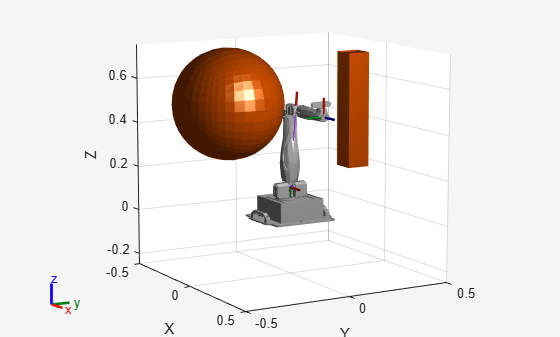# isMotionValid

Check if path between states is valid

Since R2021b

## Syntax

``[isValid,lastValid] = isMotionValid(manipSV,startConfig,goalConfig)``

## Description

example

````[isValid,lastValid] = isMotionValid(manipSV,startConfig,goalConfig)` checks if the path between two states is valid by interpolating between states using the state validator `manipSV`. The function also returns the last valid state along the path `lastValid`.```

## Examples

collapse all

Generate states to form a path, validate motion between states, and check for self-collisions and environmental collisions with objects in your world. The `manipulatorStateSpace` object represents the joint configuration space of your rigid body tree robot model, and can sample states, calculate distances, and enforce state bounds. The `manipulatorCollisionBodyValidator` object validates the state and motion based on the collision bodies in your robot model and any obstacles in your environment.

Use the `loadrobot` function to access predefined robot models. This example uses the Quanser QArm™ robot and joint configurations are specified as row vectors.

```robot = loadrobot("quanserQArm",DataFormat="row"); figure(Visible="on") show(robot); xlim([-0.5 0.5]) ylim([-0.5 0.5]) zlim([-0.25 0.75]) hold on```Configure State Space and State Validation

Create the state space and state validator from the robot model.

```ss = manipulatorStateSpace(robot); sv = manipulatorCollisionBodyValidator(ss,SkippedSelfCollisions="parent");```

Set the validation distance to `0.05`, which is based on the distance normal between two states. You can configure the validator to ignore self collisions to improve the speed of validation, but must consider whether your robot model has the proper joint limit settings set to ensure it does not collide with itself.

```sv.ValidationDistance = 0.05; sv.IgnoreSelfCollision = true;```

Place collision objects in the robot environment. Set the `Environment` property of the collision validator object using a cell array of objects.

```box = collisionBox(0.1,0.1,0.5); % XYZ Lengths box.Pose = trvec2tform([0.2 0.2 0.5]); % XYZ Position sphere = collisionSphere(0.25); % Radius sphere.Pose = trvec2tform([-0.2 -0.2 0.5]); % XYZ Position env = {box sphere}; sv.Environment = env;```

Visualize the environment.

```for i = 1:length(env) show(env{i}) end view(60,10)```Plan Path

Start with the home configuration as the first point on the path.

```rng(0); % Repeatable results start = homeConfiguration(robot); path = start; idx = 1;```

Plan a path using these steps, in a loop:

• Sample a nearby goal configuration, using the Gaussian distribution, by specifying the standard deviation for each joint angle.

• Check if the sampled goal state is valid.

• If the sampled goal state is valid, check if the motion between states is valid and, if so, add it to the path.

```for i = 2:25 goal = sampleGaussian(ss,start,0.25*ones(4,1)); validState = isStateValid(sv,goal); if validState % If state is valid, check motion between states. [validMotion,~] = isMotionValid(sv,path(idx,:),goal); if validMotion % If motion is valid, add to path. path = [path; goal]; idx = idx + 1; end end end```

Visualize Path

After generating the path of valid motions, visualize the robot motion. Because you sampled random states near the home configuration, you should see the arm move around that initial configuration.

To visualize the path of the end effector in 3-D, get the transformation, relative to the base world frame at each point. Store the points as an xyz translation vector. Plot the path of the end effector.

```eePose = nan(3,size(path,1)); for i = 1:size(path,1) show(robot,path(i,:),PreservePlot=false); eePos(i,:) = tform2trvec(getTransform(robot,path(i,:),"END-EFFECTOR")); % XYZ translation vector plot3(eePos(:,1),eePos(:,2),eePos(:,3),"-b",LineWidth=2) drawnow end```## Input Arguments

collapse all

Manipulator state validator, specified as a `manipulatorCollisionBodyValidator` object, which is a subclass of `nav.StateValidator` (Navigation Toolbox). The state validator contains properties that determine the behavior of this function and `isStateValid`.

Initial robot configuration, specified as an n-element row vector of joint positions for the `rigidBodyTree` robot model. n is the number of nonfixed joints in the robot model.

Data Types: `double`

Desired robot configuration, specified as an n-element row vector of joint positions for the `rigidBodyTree` robot model. n is the number of nonfixed joints in the robot model.

Data Types: `double`

## Output Arguments

collapse all

Valid states, returned as an m-element logical column vector.

Data Types: `logical`

Final valid state along each path, returned as an n-element row vector or m-by-n matrix. n is the number of nonfixed joints in the robot model.. m is the number of paths validated. Each row contains the final valid state along the associated path.

Data Types: `single` | `double`

## Version History

Introduced in R2021b